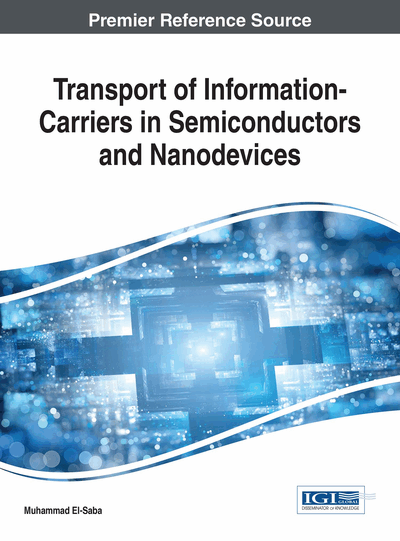# Semiclassical Transport Theory of Charge Carriers, Part I: Microscopic Approaches

DOI: 10.4018/978-1-5225-2312-3.ch002
Available
\$33.75
List Price: \$37.50
10% Discount:-\$3.75
TOTAL SAVINGS: \$3.75

Top

## 1. Overview And Chapter Objectives

The semiclassical approach is suitable for semiconductor devices whose feature dimensions are much greater than the mean free path between collisions (L >> λe). In silicon devices, where the electron mean free path λe ~100Ǻ, this approach can be safely used for devices having feature size L>0.1 μm. It helps us to calculate the main transport parameters (such as the charge carrier mobility, conductivity, etc.) and so to determine the electrical, thermal, magnetic and optical characteristics of a given semiconductor device.

In classical statistical mechanics, the state of a particle is completely defined at a certain time t as a point in a space of six coordinates, called the phase-space (sometimes called the μ-space). The six coordinates of the phase-space are the position and momentum coordinates (x, y, z, px, py, pz) as shown in Figure 2. Physically, it is not possible to do so in large systems, where the number of particles is in the order of 1022 particle per cm. Then one has to use the concept of probability or the distribution function of the system under certain conditions, like the condition of thermal equilibrium1. Therefore, we usually speak about the so-called distribution function, which expresses the average number of particles at a certain position in the phase-space. The distribution function is often written in either of the following forms:f(x,p, t) or f(x,v, t) or f(x,k, t)where:

(1a)(1b)(1c)

Evidently the momentum p and velocity v of a classical particle are interrelated by: p= mv. Also, the momentum of a semiclassical particle is related to the wave vector k by the De Broglie relation: p= h/λ = ħk. Note that, we may indifferently use v, k or p, as second argument of the carrier distribution function, in this book.

The semiclassical transport theory is based on the ensemble concept and the Boltzmann transport equation (BTE), which describes the evolution of the distribution function f(x, k, t) of a gas of particles under nonequilibrium conditions. The ensemble concept shows that a macroscopic observation is consistent with a very large number of microscopic configurations (particles), which are represented by points in the phase space. The ensemble therefore is basically represented by a normalized density distribution function f(x, k, t) in the phase-space.

## Complete Chapter List

Search this Book:
Reset